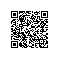# 题解 P3717 【[AHOI2017初中组]cover】

#include<bits/stdc++.h>
using namespace std;
bool a;                                  //数组开的有点大哈，开到200就足够了。不过想要标记，就要是bool型的。
int main()
{
int n,m,r,sum=0;                                 //sum为后面用的计数器。
int j=0,k=0,i=0;
int x,y;                                           //i,j,k,x,y作为控制循环次数的东东。
cin>>n>>m>>r;                                    //输入数据
for(i=0;i<m;i++)                                 //第一遍标记###看好括号###
{
cin>>j>>k;                                   //对于每一个灯塔的坐标进行输入
a[j-1][k-1]=1;                               //标记灯塔位置
/*这里要说一点，灯塔坐标给的和数组定义的不太一样。
数组的0是第一位。而灯塔的坐标是从1开始的，所以要将坐减一。
我第一次就是这么被坑的。*/
for(x=0;x<n;x++)                             //第一次搜索开始
{   for(y=0;y<n;y++)
{
if((x-j+1)*(x-j+1)+(y-k+1)*(y-k+1)<=r*r)//圆的方程：(x-a)²+(y-b)²=r²
{
a[x][y]=1;                         //标记被搜到的。
}
}
}                                            //说实话这个括号和上面“第二次搜索开始“后面的都不用加。
//为了清楚，我现在加上了。
}                                                //好了，现在搜完第一次了
//开始统计。第二次搜索，并计数
for(i=0;i<n;i++)                                 //全图走一遍。
{   for(j=0;j<n;j++)
{
if(a[i][j]==1)
{
sum++;
}
}
}
cout<<sum;                                       //输出
return 0;                                        //程序拜拜。
}使用钉钉扫一扫加入圈子
+ 订阅